# Known Issues with using POST and IF??Hello everyone,
@Misbah @JaredDolich @rob_marshall

If you know there is an issue with using POST with IF then please let us know, if you don't please read on.

Context: We would like to move a value from the current period in a line item to another line item x periods ahead under a certain condition.

IF Condition is satisfied
THEN POST(Source, x)

ELSE 99

If we don't use the condition, the POST function produces the results as expected (see below)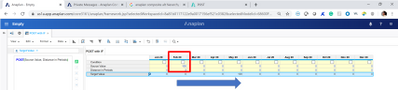If we add the IF condition based on the condition line item, POST doesn't perform as expected. It doesn't "move" the Source Value  and the formula resolves to 0 in the same period (see below)As a side note, I tried to use a numeric condition and the results were the same.

Finally, when I hard code TRUE as the condition, the POST function works (see below)Does anyone have an explanation for this behavior? even if the explanation is this is a bug.

Thanks

Tagged:

•If you drill down in May it will not show the condition is met and will return 99.

In Feb the Condition is met, but the time does not go back that far, so you are getting zero.

I believe the formula is working as it is written, and you need additional logic to make it work as you want it to.

I can try to mock up an example if that would be helpful.

•Not that I am aware of but it should work. I didn't find any constraints as well on Anapedia that  says it can not work along with other expression. Will create an example and check

•Hi @jasonblinn
Thank you

Why are you saying in Feb "time does not go back that far"? We should not be looking back, we should be looking forward 3 periods.
However, I think you are onto something because when I checked Condition in May, the Source value (100) appeared in May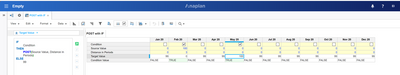Unlike OFFSET/LAG/LEAD, for example, this is how I "translate" the POST formula -
IF Condition THEN POST(Source Value, Distance in PeriodsELSE 99 In the FEB Time Period

IF Condition[FEB] is TRUE
THEN POST(Source Value[FEB], 3 Periods in the future [May])
ELSE return 99

This is how I see the difference between OFFSET (pull) and POST (push)So I don't think the formula is working as it is written (Unless my understanding is off).

Also, regarding if the condition is met or not, I added the line Item Condition Value and it shows TRUE for February, so what gives?

•Hey @Misbah

I didn't find any constraint either, that's why I'm thinking either something is wrong or I don't understand how the formula should work. either way, I need answers 😁

•For Feb: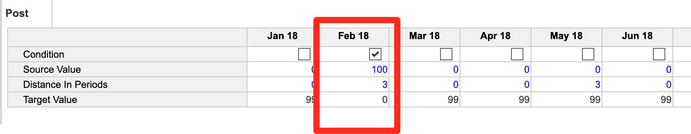IF Condition[FEB] THEN POST(Source Value, Distance In Periods)[Nothing that meets this critera] ELSE 99

Since there is nothing in the Post logic that is telling it to land in FEB then your formula is showing 0

For May: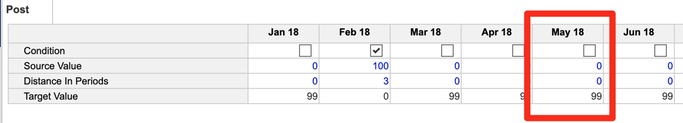IF Condition[MAY] THEN POST(Source Value, Distance In Periods)[FEB] ELSE 99

Since in May the condition is not met then it is jumping to the 'ELSE 99' Argument of the formula.

Using this formula, you would need to check the condition in May: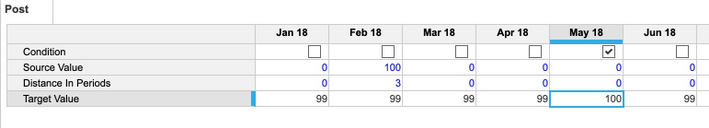IF Condition[MAY] THEN POST(Source Value, Distance In Periods) ELSE 99

The condition portion of the formula does not get considered in the post.

If you could have them check the condition in the month that it should be displayed in, that would be the easiest I believe, otherwise, you need to likely do some staging move this data in the way you are wanting.

I hope this helps! I am happy to try to mock this up over the next day or so if that would be helpful using the different staging lines.

Jason

•Thank you very much @jasonblinn

I think I see where you and I differ.

When you say ....

1. IF Condition[FEB] THEN POST(Source Value, Distance In Periods)[Nothing that meets this critera] ELSE 99
Well, the criteria is met. Condition[Feb] is TRUE
So I don't understand this point.
2. "Since there is nothing in the Post logic that is telling it to land in FEB then your formula is showing 0"
The POST logic should be telling it to land in MAY, not in FEB. Why do you say FEB? I'm in FEB (starting point) asking that the value lands in MAY.
3. If you could have them check the condition in the month that it should be displayed in, that would be the easiest I believe, otherwise, you need to likely do some staging move this data in the way you are wanting.
If we wanted to check the condition in MAY, we would use OFFSET/LAG, but since -according to the requirement- when we are in MAY I don't know how many periods we should go back, we use POST in the source because in FEB I know exactly how many periods I need to move.

Am I not correct when saying the POST works differently than OFFSET/LAG?

I think what is causing the confusion is that the POST on its own is working fine.
The POST with a hardcoded TRUE also works, It is only when I'm asking that the condition be checked dynamically.

•I think we are saying the same thing, but the issue is with how the IF Condition part is working. Since that is in no way tied to the Post portion of the formula it is looking at the month in which it falls (aka its ITEM(TIME)). The Condition for FEB impacts the Target Value in FEB. The Condition for MAY impacts the Target value for MAY. the Condition for FEB does NOT impact the Target value for MAY.

1. Since you have condition in FEB checked, then the Target value for FEB is 0 since there is nothing that gets posted into the month of FEB. The Condition for FEB only impacts the Target Value for the Month of FEB, so the formula is working as it is written.

2. The post logic is telling it to land in MAY but it will only land there if the Condition is checked in MAY otherwise it will show 99. Since you are seeing 99, then the formula is working as it is written.

3. Correct, This is where you would need to stage this. You will probably want staging lines that say: IF Condition then Source Value ELSE 0... And one for the Distance in periods, and then you can base your POST formula on those staging line items.

The POST is working properly, but the formula with how it is written is not allowing it to work the way you want it to.

Jason

•Firs of all thanks for discovering this and sharing this with us

I am leaning towads @jasonblinn explantion. Here boolean is supposed to be checked against each month and if it is not checked for a specific month and at the same time POST function is supposed to push values to that particular month, numbers are not going to get populated in that month.

Scenario 1 Below:

Jan is checked but April is not checked in Boolean condition

Number of periods (3) expects numbers to be pushed to April

Since April is not checked POST throws ELSE output which is zero in this case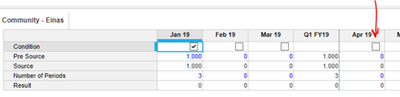Scenario 2 Below:

Jan is checked and April is checked too in Boolean condition

Number of periods (3) expects numbers to be pushed to April

Since April is checked POST pushes the values (1000 )from Jan to April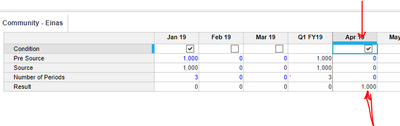Scenario 3 Below:

Jan is not checked but April is checked in Boolean condition

Number of periods (3) expects numbers to be pushed to April

Since April is checked POST throws ELSE output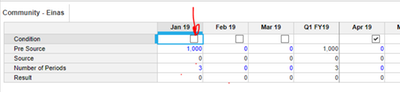Workaround:  We have to split the IF statement in a diff line item

Bottom line: There should be constraints in the formula that we can't use such IF statements with POST

•Thanks @Misbah

In my opinion, to check the boolean of the target month doesn't make sense, and wouldn't fulfill the "business case/requirement". That boolean represents a certain condition,  for example, initiate a money transfer in Feb so it would arrive at the other account in May - based on the number of periods.
If we check May as well then we are saying initiate a transfer in May too. that doesn't agree with the requirement.

Also, if you think of this as a User Story- as a user I want to be able to initiate a money transfer in a certain period and based on the bank or the transfer method, account for that money in the period when it should arrive.
As the user, I should expect to check the boolean in say Feb only and have everything else automatically calculated.

So, my conclusion - unless someone convinces me otherwise -  is that this is a bug or best case scenario a constraint should be added to the POST function technical documentation stating that it can't be used with IF.

•I have a use case where I am currently doing some capacity planning and want to check a condition in the week that I want to plan this capacity in, and it then brings it into the correct week. It is working exactly as intended right now. Point being, there are tons of business cases, and if they were to change how it is currently working, then anyone who is using the current functionality would need to update their formulas. If they were to change how IF with POST works, then I would have to stage it just like you do now.

There are a ton of things in Anaplan that need to get staged for them to work for each individual business use case, and this is one of those cases. Here is an example of how you can quite simply make this work based on your user story.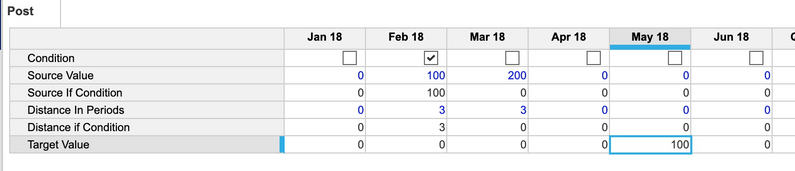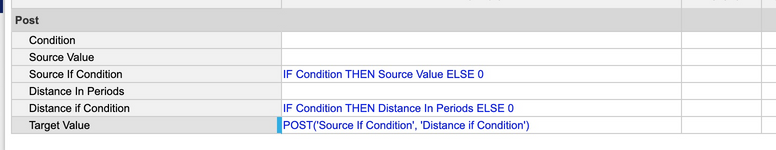As you can see, I check the condition in my source month, which then populates my IF line items which get posted.

Jason

•I tend to be with you here that this is un-natural.
I think what happens is that when the condition is false, then POST doesn't execute, and it seems Anaplan does not run this function period by period but as a whole.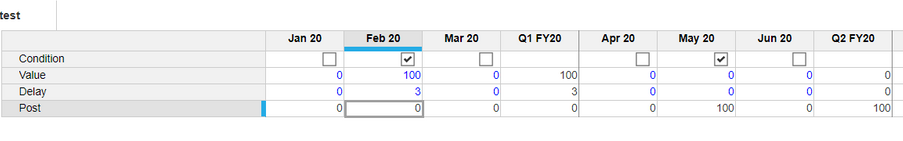•that makes sense.

I know we are able to find workaround but I can’t justify the way it works as “normal”.

That’s the difference.

•Hi @einas.ibrahim

You have run into a fundamental behaviour for Anaplan Calculation, I'll go into that a bit and then show a couple of solutions (one good, one not so good).

Background
A formula is made by joining together functions, and for all but a few functions:

The evaluation of a function:
1. occurs at the target point*
2. without knowledge of the formula around.
Now, there are exceptions to point 1, but never to point 2. These exceptions occur with certain coordinate** transformations (offsets, post, currentversion, cumulate, … ), textlist, and rank functions - for these functions the first parameter, the value, can itself be an expression and this expression is evaluated at a different point.

In case of offsets, post, and cumulate, this moving of the point of evaluation occurs along a specific dimension as a coordinate shift. Let's work through the formula:
IF Condition THEN POST(Source Value, Distance In Periods) ELSE 99
• at point (May 20) the condition is FALSE, so return 99
• at point (Feb 20) the condition is TRUE, so return POST(Value, Offset) - this will return the sum of all values that have an offset targeting Feb 20. Note that this will include all values, regardless of whether the condition is true or not at the source point.

I think @jasonblinn and @Misbah have given explanations of this previously.

Solution
Now, with all that background, let's get to the solution. I believe that you only want to only post the value if the condition is true, and if the condition is not true we want to add the fill value.

Jason nearly had this. The missing piece is that the first and second parameters to post can be expressions, and these expressions will be evaluated for all Time coordinates. It is at this point that values that have the correct offset amount are 'posted' to the 'target' cell.

I have two slightly different solutions, first:

POST(IF Condition THEN Source Value ELSE 99, IF Condition THEN Distance In Periods ELSE 0)

This is essentially moving Jason's logic inside the POST. I wouldn't do this though, as this is more computationally heavy than the following:
IF Condition THEN POST(IF Condition THEN Source Value ELSE 0, Distance In Periods) ELSE POST(IF Condition THEN Source Value ELSE 0, Distance In Periods) + 99
This formula is saying 'if the condition is true for the current point then get any values that are posted to it, and otherwise get values that are posted to the current point and add 99. This could be rewritten as follows by moving the repeated POST out of the IF
POST(IF Condition THEN Source Value ELSE 0, Distance In Periods) + IF Condition THEN 99 ELSE 0
This is easier to understand, but slightly slower computationally (we always do an addition, rather than only doing it if necessary).

Conclusion
There is no bug/issue wit
h POST, IF or any combination of POST and IF.
The solution to the problem requires an understanding of how a formula f is evaluated at any given point p, and also of how special functions like POST allow you to reference source data and even calculate at a point other than p.

Terminology

* Point: the position of the cell that is being evaluated, examples:
(Jan 20, Actual, Product X)
(May 20)
** Coordinate: the position in a given axis of a point, e.g. the Time coordinates for the points above are Jan 20, May 20.

Hope this helps! 🙂 Keep modeling!
Dan
•@daniel_harman  Class!

How on earth did I miss POST(IF..........). Thank you for the deeper analysis of this issue.

•Hi @daniel_harman

Thank you very much for the detailed and deep analysis you provided.
I believe the new piece of information I got from your post - no pun intended 😊 - is that the POST() function - along with a few similar ones - calculate at a different point than the rest of the of functions or as we expect.
My question is why? --- Yes I'm like a 3 years old who keeps asking why until receiving a satisfying answer 😂

I'll dig deeper into this a bit to learn more, and I would appreciate your help if I have questions. the first being how did you come across this information?

I really appreciate everybody's participation in the discussion, it was most helpful

Update from L3

I shared my question with Anaplan Support and they escalated the issue to L3. I received the L3 response yesterday which stated
"I have received a response from the Development team and they have determined that this is not a bug with the platform and the behaviour you have observed is working as designed. In your example, as the condition is not met in the May 20 cell, the formula will return 99 for that cell which overrides the POST coming from the Feb 20".

They didn't provide any more explanation. Thanks @jasonblinn for your examples.

I wasn't really looking for workarounds as much as being very curious as to why POST() is not doing what is expected at first glance.

I believe if the part about the way the function(s) calculates is explained in Anapedia, it would provide more clarity.

Again, I really appreciate the discussion and the participation

•Why is a good question. I wouldn't say that functions calculate in an unexpected way, rather there are different categories of functions that provide different capabilities to the modeller.

It comes down to wow Anaplan formulas work, so let's consider something basic and familiar.

Given:
List X with items x1, x2, x3, and top level xTotal
Line Item A, dimensioned by X and Quarters, values:

```|        | Q1 | Q2 | Q3 | Q4 |
| x1     | 1  | 2  | 3  | 4  |
| x2     | 1  | 1  | 2  | 3  |
| x3     | 1  | 2  | 4  | 8  |
| xTotal | 3  | 5  | 9  | 15 |```

Line item B, dimensioned by Quarters, formula = A * ( 9 + 1 )
Then the values of B are:

```| Q1 | Q2 | Q3 | Q4  |
| 30 | 50 | 90 | 150 |```

Why? Well we work through the calculation step by step. First we're doing a multiplication. All that multiplication does is multiply two numbers, it doesn't need to know where they come from! Next we need to get those two input numbers to multiply. The first number comes from a line item reference, and the second is given by adding 9 and 1, that is 10.

The line item reference uses a few simple rules - we match coordinates for all axes where possible, and if a dimension is missing then we use the top level. So in this case when we evaluate the formula at point (Q1) we get the value from A at (Q1, xTotal), which is 3.

This is obviously very different to an Excel way of operating where you provide a different formula cell by cell.

Most functions work in this manner - they don't need to know where the values come from - we can call these 'Value' functions. These include Logic, Arithmetic, Call Centre functions, and more...

Now sometimes we don't want to match all the coordinates, for various reasons. That is where the other classes of functions come in:

 Expression Allowed Expression not allowed Cumulate, Decumulate, HalfYearToDate, MonthToDate, QuarterToDate, WeekToDate, YearToDate- cumulate over a given axis (time) Collect- a bit like a line item reference but adding the line item itself to a dimension of the result, the standard reference rules still apply though Lag, Lead, Next, NextVersion, Offset, Previous, PreviousVersion- offset coordinate on a given axis (time or versions), all other coordinates of the point are unchanged HalfYearValue, MonthValue, QuarterValue, WeekValue, YearValue- get source value at a given ancestor level (time) Profile, Post, Spread- sum up values on a given axis (time) where the source values are at an offset coordinate at which the defined offset amount returns the result coordinate (not quite a 'push', as we've seen) MovingSum, TimeSum- sum up values over a specified time range IsFirstOccurrence- first occurrence of value on a given axis (a list) Sum, Min, Average, FirstNonBlank, Textlist, ...- dimensional transformation - collect the points of the source for which the relation value is the coordinate for a specific dimension in the target point, then combine the values of the source at these points to produce a single value (e.g. combine using addition for Sum) Textlist- concatenate expression over an axis (a list) Select, Lookup- dimensional transformation - pick a point in the source by specifying a coordinate for a specific dimension, either by a static selection (SELECT) or by using the lookup relation value at the target point (LOOKUP), then return the value of the source at this point. Rank, RankCumulate- rank/cumulate values over a block (Version + Time coordinates are fixed)

These functions allow us to take in data from points other than that which direct line item reference would give us, to consider previous data, or a total dataset, or map data from one space to another. Personally I find this is where the fun kicks in!

To go into POST behaviour in more detail:
POST(valueExpr, offsetExpr) evaluated at point p:

• The point p has a time coordinate, p(Time).
• Find all other points q, with time coordinate q(Time), and for all non-time axes q = p, where the offset on the time axis between q(Time) and p(Time) is equal to the evaluation of offsetExpr at q.
• Evaluate valueExpr at all these q, and sum them up.

You can see in this manner of expressing, it isn't that the values are pushed, so much as fetched when they match the condition such that they would push. Subtly different!

That got a little technical in terminology again, sorry, it's hard to describe the precise behaviour without getting technical 🙂
As to how I got this information - a lot of experimentation over a long time!

Dan